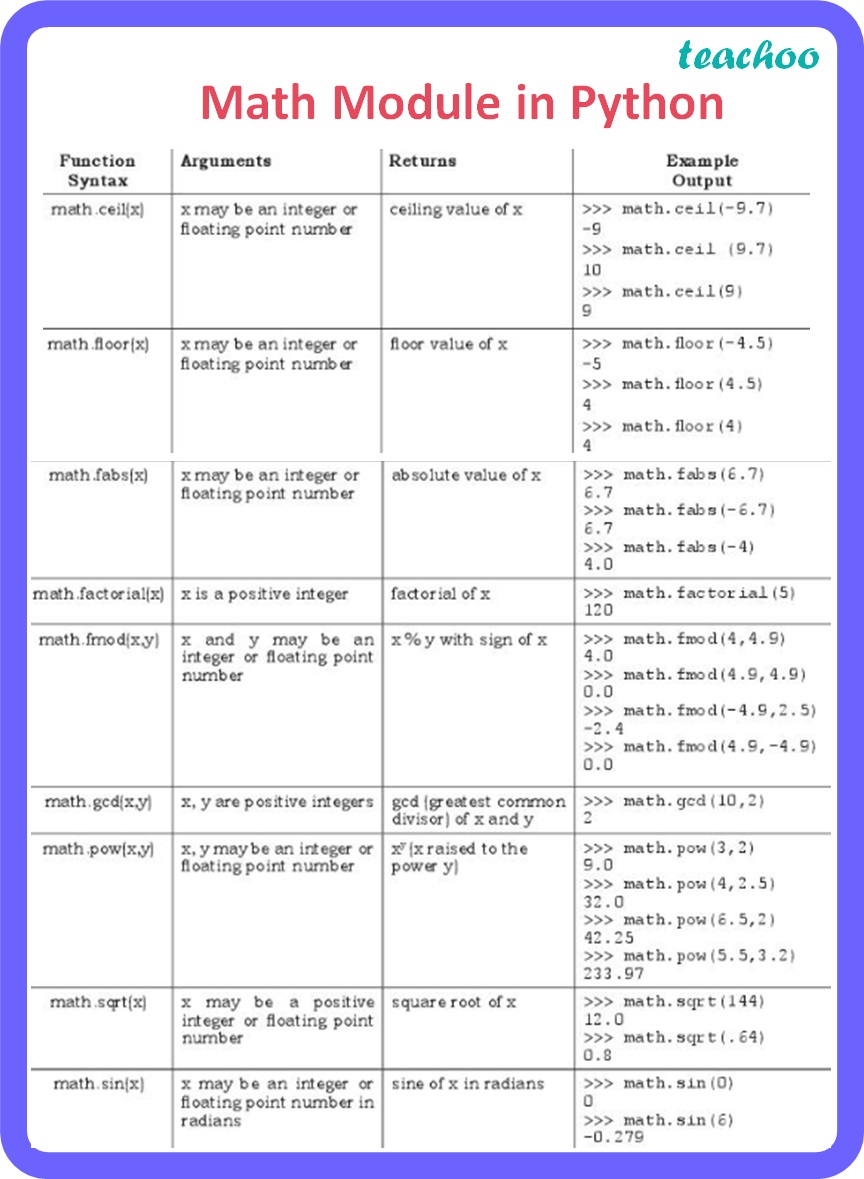Concepts

Computer Science - Class 11
Chapter 7 Class 11 - Functions

## Math Module in Python

It is a built-in module which contains different types of mathematical functions.

In order to use the math module we need to import it using the following statement:

import math

Most of the functions in this module return a float value

Commonly used functions in math module are given below:Learn in your speed, with individual attention - Teachoo Maths 1-on-1 Class